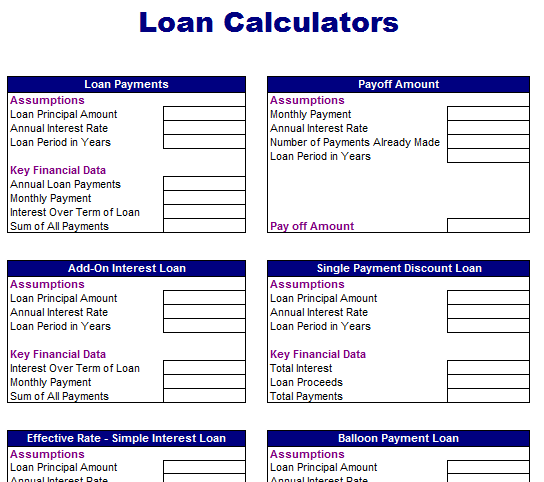# Common Loan Calculator

Loan is amount of money that can be got from bank or financial institution on some interest rate. Principal amount, interest rate and date of payment are some main factors of loan. Whenever you take loan, the lender charges some interest after considering inflation rate to make profit. There are different types of loans that have different interest rate and compounding period. Often compounding of interest can higher the amount of interest you pay on the loan. It is necessary to calculate compound interest on an auto loan so that you can know how often interest compounds and the annual interest rate. Usually, financial organizations provide you complete details of loan and interest rate but you can personally calculate it.

Tips for Common Loan Calculations

If you are worried about the total amount of interest then you have to use following useful tips to perform some common loan calculations:

• In first step, find annual rate and divide it with total times of each year compounding to get the periodic rate. For instance, if you have an loan that compounds interest daily and carries an annual rate of 18 percent divide 0.18 by 365 to find a daily rate of 0.0005.
• Add 1 to the periodic rate such as 1 + 0.0005 = 1.0005.
• Raise the answer to the power of the number of days that interest accrues before you make a payment. For example, if you make a payment every 30 days then raise 1.0005 to the 30th power to get 1.015109259.
• Subtract 1 from this answer to get rate of interest such as after subtracting 1 from 1.015109259 to get 0.015109259.
• Multiply the result by the balance of your loan to find interest compounding on your auto loan. According to current example, if you have \$20,000 remaining to pay on your loan then multiply 1.015109259 by \$20,000 to find \$302.19 of interest accrues.

Here is preview of a worthy Common Loan Calculator that offers all common loan related calculations,Fixed-rate Loan

Fixed-rate loan can be easy to pay because it carries the same interest rate over the whole life of loan. It can be easier to your budget because each monthly payment of this loan remains constant. These are different types of fixed-rate loans like mortgages, car loans and residential or home loans. If you want to calculate the monthly payment and duration of this loan then you can follow below mentioned procedure:

• Consider the total amount of money you are borrowing for example, \$50,000. “Name this digit such as you can call this \$50,000 like A.” Convert the annual interest rate from a percentage to a decimal by dividing by 100. For instance, if the annual interest rate is equals to 8 percent then you would divide 8 by 100 to get 0.08.
• Now calculate the monthly interest rate by divide the annual interest rate by 12 and call this M. For instance, you can divide 0.08 by 12 to get M = 0.007.
• It is time to calculate the number of monthly payments that you will make over the life of the loan by multiplying the years you will take to repay the loan by 12 and call this P. For instance, if the total life of loan is 20 years then you can multiply 20 by 12 to get p = 240 total payments.
• Put the values of A, M and P in the below given equation to compute the monthly payment on the fixed-rate loan:
• Monthly Payment = (M / (1 – (1+M) ^ – P)) * A
• You can plug \$50,000 for A, 0.007 for M and 240 for P, so your expression would be (0.007/(1-(1+0.007)^-120))*50,000, which would simplify to a monthly payment of \$617.26.